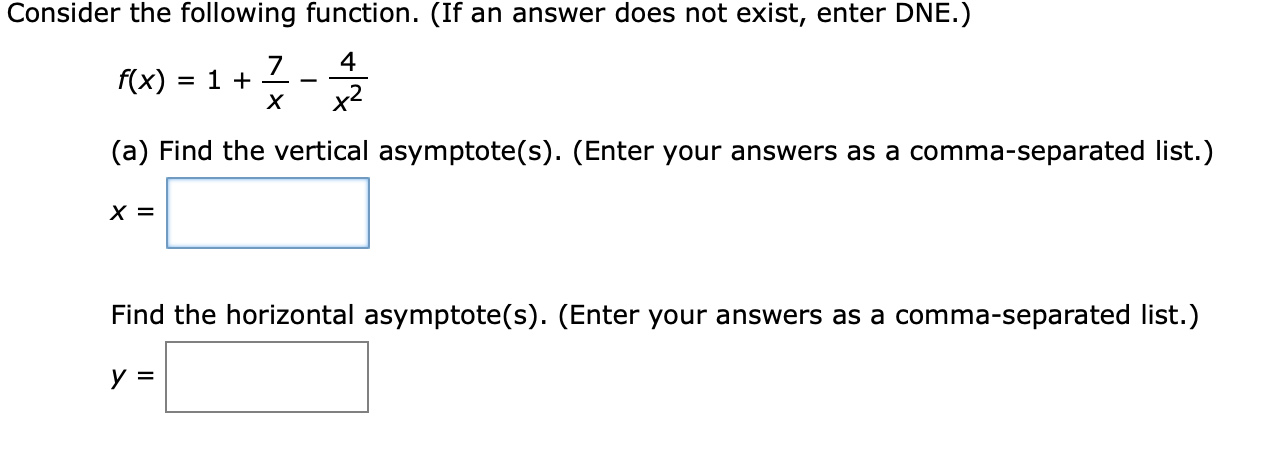# Consider the following function. (If an answer does not exist, enter DNE.)7= 1 +хf(x)x2(a) Find the vertical asymptote(s). (Enter your answers as a comma-separated list.)х 3Find the horizontal asymptote(s). (Enter your answers as a comma-separated list.)y =

Question
110 viewshelp_outlineImage TranscriptioncloseConsider the following function. (If an answer does not exist, enter DNE.) 7 = 1 + х f(x) x2 (a) Find the vertical asymptote(s). (Enter your answers as a comma-separated list.) х 3 Find the horizontal asymptote(s). (Enter your answers as a comma-separated list.) y = fullscreen
check_circle

Step 1

Consider the given function.

Step 2

Rewrite the above function as,

Step 3

Part (a)

To find the vertical asymptotes of the function, ...

### Want to see the full answer?

See Solution

#### Want to see this answer and more?

Solutions are written by subject experts who are available 24/7. Questions are typically answered within 1 hour.*

See Solution
*Response times may vary by subject and question.
Tagged in

### Calculus